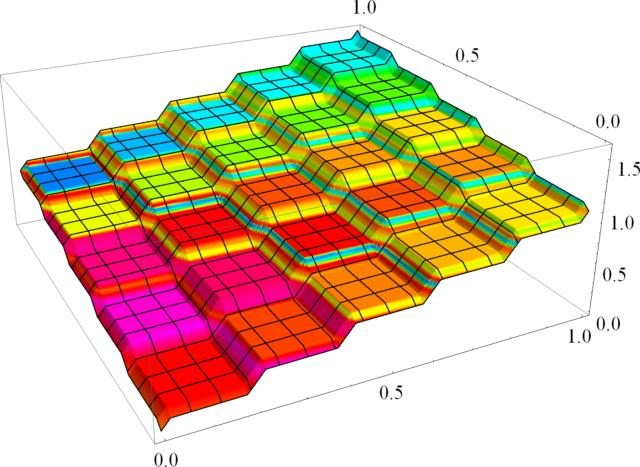© | << < ? > >> | Dror Bar-Natan: Talks:

# Kolmogorov's Solution of Hilbert's 13th Problem

### The Graduate Student Seminar Department of Mathematics, University of Toronto September 30, 2009

Are there any truly new continuous functions of two variables, beyond addition and functions of one variable? Well, multiplication isn't one, for multiplication is a simple composition of addition and of functions of just one variable: xy = exp(log x + log y). Powers and logarithms wouldn't do either, as xy = exp(exp(log y + log log x)) and logx y = exp(log log y + (- log log x)). Trig functions won't do, they are functions of one variable. Maybe Bessel functions? Anybody has a clue what they are? Anyway, according to Kolmogorov and Arnol'd, we need not worry, for the following amazing theorem holds true:

Theorem. Any real-valued continuous function on a compact set in Rn is a finite composition of (several instances of) the binary function "+" and of single-variable continuous functions.

On top of being a beauty, this theorem also resolves the 13th of Hilbert's famed 23 problems, in which he presented a certain specific function of three variables and asked if it can be re-written as a composition of continuous functions of two or less variables. Well, our theorem does a lot better.

Ok, if you can't make it to the lecture or if you want to do some work in advance, just do some exercises!More under Academic Pensieve: 2009-09: Mathematica Notebooks: Hilbert13th.